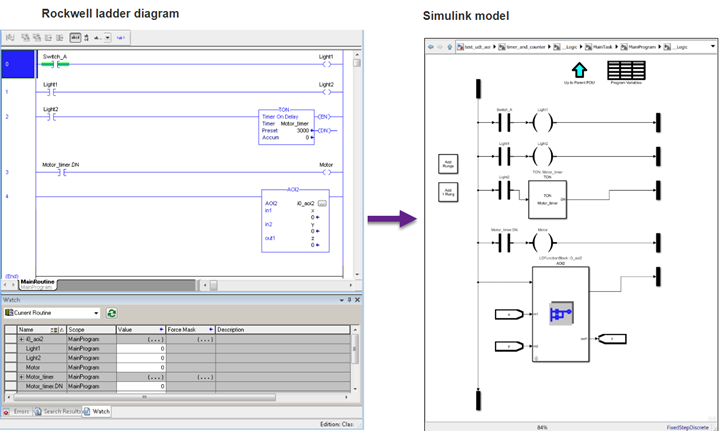Main Content

# Ladder Diagram Integration

Import, simulate, and validate your existing ladder diagrams. Model, simulate, and generate code using the provided Ladder Diagram blocks and functions.

Ladder Diagram is a graphical programming language that you use to develop software for programmable logic controllers (PLCs). It is one of the languages that the IEC 61131 standard specifies for use with PLCs. A program in ladder diagram notation is a circuit diagram that emulates circuits of relay logic hardware. The underlying program uses Boolean expressions that translate readily to switches and relays.

With Simulink® PLC Coder™, you can use ladder import to import ladder diagrams created with Rockwell Automation® IDEs, such as RSLogix™ 5000 and Studio 5000 into the Simulink environment as a model. You can create ladder diagrams in the Simulink environment as a model.

After importing or creating the ladder diagram models, you can:

• Edit the imported Ladder Diagram model from within Simulink by using the `plcladderlib` library.

• After importing the ladder diagram code into Simulink, simulate it.

• Generate C code from the imported ladder diagram and integrate the code into your existing C language-based simulation environments.

• Generate ladder diagram code from the imported ladder diagram and integrate the code into your Rockwell Automation IDE. You cannot generate structured text code from the Ladder Diagram blocks.

• Generate PLC testbench code for the imported ladder diagram and verify the code in your Rockwell Automation IDE.## Apps

 Simulink PLC Coder Generate structured text and ladder diagram code from Simulink models, Stateflow charts, and MATLAB functions

## Functions

expand all

 `plcimportladder` Import ladder diagram into a Simulink subsystem `plcgeneratecode` Generate structured text or ladder diagram (L5X) for the atomic subsystem `plcladderinstructions` Lists ladder instructions identified by Simulink PLC Coder
 `plcladderlib` Open the Simulink PLC Coder Ladder Library
 `plcgeneraterunnertb` Generate L5X test bench code for specified AOI Runner block and AOI name
 `plcladderoption` Get or set parameter values associated with Ladder Diagram models `plcloadtypes` Load the data types for Simulink PLC Coder ladder models `plccleartypes` Clear the data types associated with the Simulink PLC Coder ladder models from the workspace

## Blocks

expand all

 ONS One Shot OSR One Shot Rising OSF One Shot Falling XIC Examine If Closed XIO Examine If Open OTE Output Energize OTL Output Latch OTU Output Unlatch
 TON Timer On Delay TOF Timer Off Delay RTO Retentive Timer On CTU Count Up CTD Count Down RES Reset
 JMP Jump LBL Label TND Temporary End AFI Always False NOP No Operation MCR Master Control Reset
 ADD Add inputs SUB Subtract inputs MUL Multiply inputs DIV Divide one input by another CPT Evaluate expression AND Bitwise AND OR Bitwise OR NOT Bitwise NOT NEQ Not Equal To EQU Equal To LEQ Less Than or Equal To GEQ Greater Than or Equal To LES Less Than GRT Greater than
 MOV Move CLR Clear COP Copy File FLL File Fill
 AOI Runner AOI Runner PLC Controller Suite PLC Controller PLC Controller PLC Controller Task Task Ladder Diagram Program Ladder Diagram Program Ladder Diagram Subroutine Ladder Diagram Subroutine Ladder Diagram Function Block (AOI) Ladder Diagram Function Block (AOI)
 Variable Read Variable Read Variable Write Variable Write
 Power Rail Start Power Rail Start Power Rail Terminal Power Rail Terminal Rung Terminal Rung Terminal Junction Junction
 Custom Instruction Create custom ladder logic instruction

## Topics

Supported Features in Ladder Diagram

Ladder features and elements that are supported by Simulink PLC Coder.

Import L5X Ladder Files into Simulink

Importing ladder diagram files into Simulink.

Modeling and Simulation of Ladder Diagrams in Simulink

Create Ladder Diagrams in Simulink and perform simulation.

Generating Ladder Diagram Code from Simulink

Generate Ladder Diagram code (L5X) from Simulink.

Generating C Code from Simulink Ladder

Generate C code from a Simulink Ladder Diagram Model.

Verify Generated Ladder Diagram Code

Verify generated code against the original Simulink model in your IDE using a generated test bench.

Create Custom Instruction in PLC Ladder Diagram Models

Create user-defined instruction in PLC Ladder Diagram models.

## SupportGet trial now